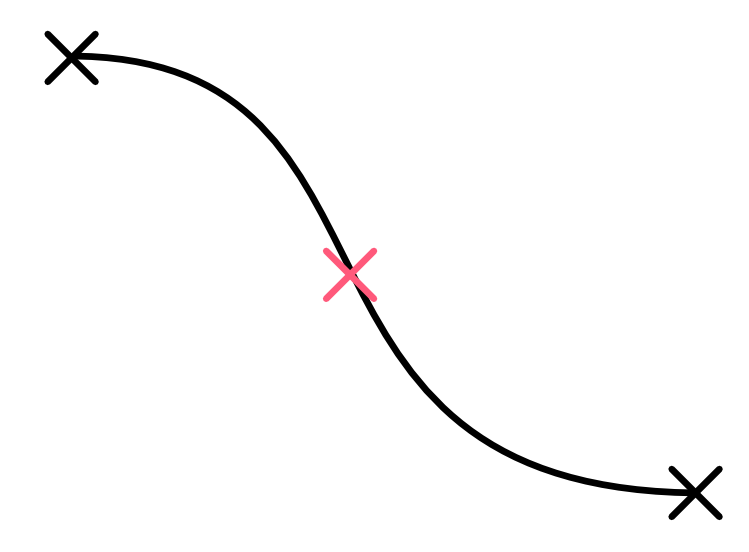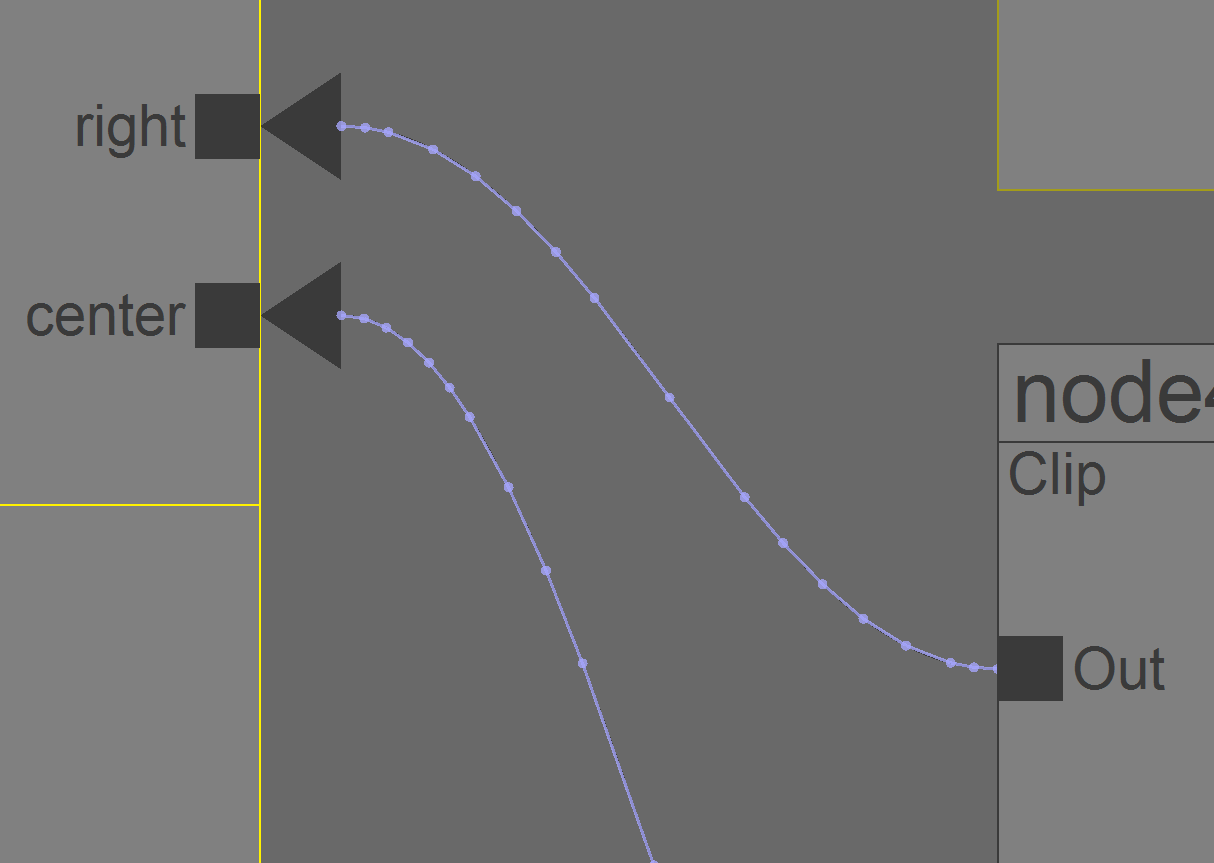### Checking whether a point lies on a Bezier curve

Introduction
It has been a long time since my last post. I was busy in the past months, and finally have time to finish dual-quaternion skinning and added some basic animation to my code base.The animation editor
In the animation tree editor, those tree nodes can be connected/disconnected, which displayed by a cubic Bezier curve. I want the user can right click the curve to disconnect the tree nodes, so checking whether a point lies on a Bezier curve is need.

Sub-dividing the curve
A cubic Bezier is defined as:

solving an analytical  solution for this is quite complicated. So I just sub-divide the curve into several line segments and stop the sub-division if the points are close to a straight line. After that we can check if the point is lying on the line segments instead. First we start at the 2 end points of Bezier curve and check whether the mid-point(the red cross) need to be sub-divided.check whether the red point need to be sub-divided
We can't simply check whether this 3 points close to a straight line because there may have an inflection point on the curve, so we need to look ahead 1 step further to see whether the 2 line segments(the blue and green lines below) are a straight line, if not, then the red point need to be sub-divided.look ahead one more point to avoid inflection point problem
Repeat these steps for each newly generated sub-divided curve segments until all the points are close to a straight line (with a threshold). The sub-divided curve may look like this:

With this approach, when we zoom into the graph, more points will get sub-divided:Zooming in will sub-divide more points

Conclusion
This short post describe a way for checking whether a point is on a cubic bezier curve by using line sub-division. In the next post, I will talk about another fun stuff when writing the animation editor.